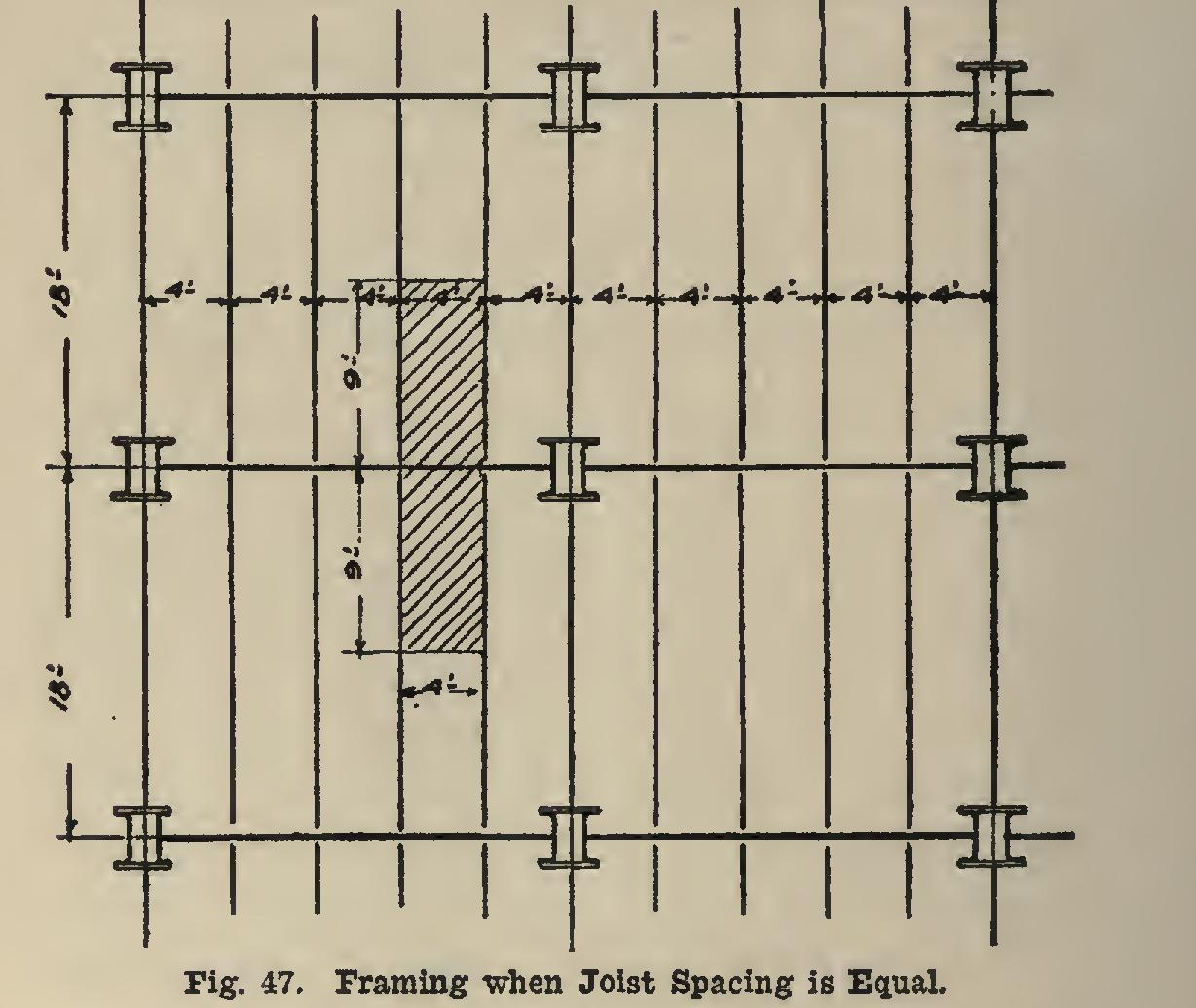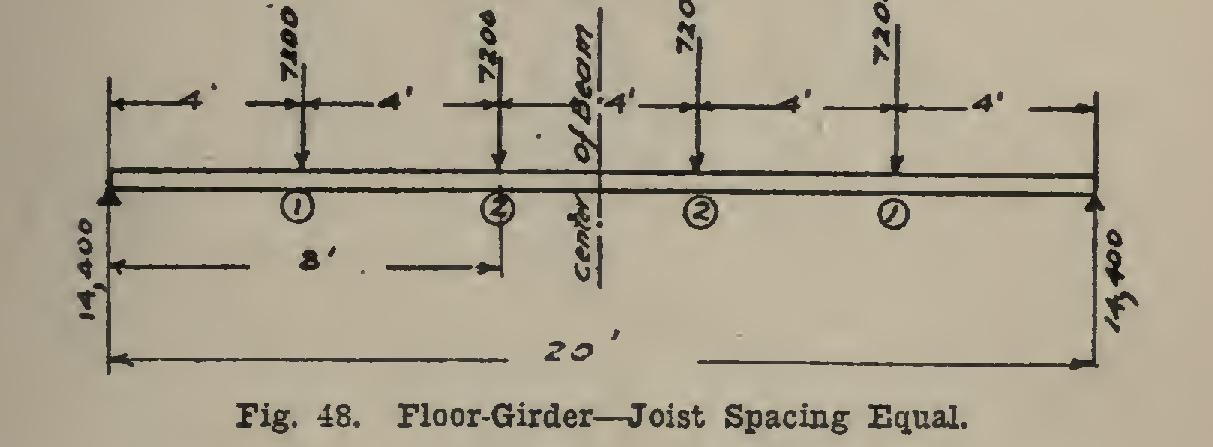Home >> Radfords-cyclopedia-of-construction-vol-4-masonry-wiring >> 325 At Load Moment to Wrought Iron 9 >> 325 at Load Moment

325 at Load Moment in Pound-Inches 17

pounds, cross-girder, beams, fig, i-beam, carry, square, beam, 15-in and required

AT LOAD MOMENT IN POUND-INCHES 17,325 39,527x4x12= 1,660,134 15,750 39,527x8ix12-17,325x5x12 = 2,992,254 20,475 39,527x131x12-(17,325x10x12+15,750x 5x12)= 3,379,374 23,625 39,527x211-x12-(17,325x18x12+15,750x 13x12+20,475x8x12)= 2,033,166 This shows that the greatest moment occurs un der the load of 20,475 pounds.

If the allowable unit-stress is 16,000 pounds per square inch, the section modulus is: By consulting (C 98), column 11, it will be seen that not even the largest I-beam is strong enough. If the loads on the floor were fixed, as in the case of heavy machinery, a unit-stress of 20,000 pounds per square inch would be allow able. The S required will then be: 5=3,379,3720,004468.97, and a 24-in. 80-pound I-beam may be used. This has S=174.0, but it is the value which comes nearest to the required 168.97.

In case the allowable unit-stress were 16,000 pounds per square inch, as required above, two I-beams riveted together would be used, or, bet ter still, a built-up girder such as is discussed in the next article.

In Fig. 46, where A-B is shown, the reaction will be as shown, and the greatest moment will occur under the 36,855-pound load; and it will be 6,083,219, which, at 16,000 pounds per square inch, requires a section modulus of 6,083, 219=16,000 = 380.1; and here, as before, two large I-beams or a built-up girder may be used.

The most difficult case has been discussed in the foregoing presentation. Usually, in prac tice, few such cases occur in building work. The floor joists or beams are usually spaced equally, and the columns are also spaced so that the cross or floor girders are of the same length through the building (see Fig. 47). This simplifies mat ters considerably, since all the beams are alike and the cross-girders are loaded symmetrically both as to position and as to weight of loads. If the floor load due to its own weight was 20 pounds per square foot, and that due to the load it was to carry was 80 pounds per square foot, then the cross-girders of Fig. 47 would be loaded as shown in Fig. 48, each load being 18X 4 x 100 =7,200 pounds. This, it will be noticed, is the amount of load that comes on the shaded area, Fig. 47. It is twice the reactions of one beam. Each reaction of the cross-girder (see page 163) is equal to 2 X 7,200=14,400 pounds.There should always be an uneven number of spaces on the cross-girder, as in Fig. 48, where there are five. If there were an even number, one floor-beam would come in the cen ter of the cross-girder (see Fig. 49), and this would (as you will find by designing the beam) require a larger cross-girder to carry the same load than would be required if the number of spaces were uneven. In cases where they are spaced as in Fig. 48, the moment will be the same under each of the beams equidistant from the center of the cross-girder; and also it will be the greatest under the beams nearest the center of the cross-girder. In the case of Fig. 48, the greatest moment is : pound-inches.The design of the small beams which carry 7,200 pounds can be made by the second method page 184). Here, if the allowable unit-stress is

16,000 pounds, C=7,200x18=129,600; and in (C 100), 12th column, is found 151,700, the nearest value that can be used. This belongs to an 8-in. 18-pound I-beam.

The cross-girder will be designed by its sec tion modulus. The required S is: S=1,036,800+16,000=64.8 ; and from column 11 (C 98), a 15-in. 50-pound I-beam is seen to be just too small. A 15-in. 55-pound I-beam will be used.

In all of the above cases the weights of the beams have been neglected, since they are less than one-tenth of the load they carry.

In practice, where a lot of joists are equally spaced along one cross-girder, it is, by some poor designers, considered that the action of the load is the same as if it were a uniform load over the entire cross-girder. The cross-girder in question will now be so designed by this method.

C=20x4x7,200=576,000 This would call for only a 15-in. 42-pound I-beam, whereas the correct design requires a 15-in. 55-pound beam—a beam 13 pounds heavier. This shows that this practice is incor rect, and should not be followed.

On pages (C 91-94) is much valuable informa tion regarding the tables of beams and their loads. When two or more letters are written together, it means that they are multiplied to gether. For example P1 means that P is multi plied by 1. If one set of letters or figures appear with a line between, it means that the top one is divided by the bottom one; as Pl/AE means that P is multiplied by 1, and the result is divided by A multiplied by E. In case a small figure is seen up near the top of the letter, as or it means that the number is multiplied by itself that number of times. That is, and The understanding of the fore going notation is essential to the person who would use (C 91-94).

In order not to have the plastering crack in buildings on account of the bending of the beam, the load must not exceed a certain amount, even if it can carry more. In (C 67-69) is given much information regarding the limits of span length and loading. In tables (C 70-74) are horizontal black lines in the columns. This signifies that only beams above that line can be used if plas tering is on their under surface. For example, on page 70, looking in the 15-in. 80-pound I-beam column, you will see that this beam can be used for spans from 12 to 24 feet, if plastering is used; and if it is not used, these may be used up to 36 feet, the loads they carry in each case varying with the span.

39. Shears and Moments in Girders. These are computed in exactly the same manner as for beams. Unless the load is a uniform one, de signing of girders by taking their entire section directly from the Carnegie Handbook is rather limited. The determination of the sizes must usually be made by design, but in case a "God frey's Handbook" is available, girders of almost any size and for any purpose can be taken directly from the tables given therein. This handbook is invaluable to the advanced worker, and is of great advantage to the beginner.